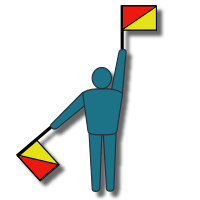# Binary Code: Codes and Secret Messages

Binary Encoder Enter some text here to be converted to binary. Basic Explanation Did you

Binary Encoder

## Basic Explanation

Did you know that everything a computer does is based on ones and zeroes? It’s hard to imagine, because you hear people talking about the absolutely gargantuan (huge) numbers that computers “crunch”. But all those huge numbers – they’re just made up of ones and zeros.

It’s kind of like the computer is made up of a bunch of lightswitches, and each lightswitch controls just one lightbulb. On or Off. One or Zero. But if you took all of those lightbulbs together, and said “Let’s make each sequence of On-and-Off represent a different number!” Well then, you could get some pretty large numbers.

What do I mean by sequence? Let’s say you had two lightswitches. There are four different ways we could flip those switches:

Both Off

First Off, Second On

First On, Second Off

Both On

Binary Code takes each of those combinations and assigns a number to it, like this:

Both Off = 0

First Off, Second On = 1

First On, Second Off = 2

Both On = 3

## Intermediate Explanation

Another way of thinking about it is this: let’s give each lightbulb a point value. We’ll say the first lightbulb is worth two points, and the second one is worth one point. Now take a look at the combinations:

Both Off = 0 + 0 = 0

First Off, Second On = 0 + 1 = 1

First On, Second Off = 2 + 0 = 2

Both On = 2 + 1 = 3

If we added in another lightbulb, we would make it worth twice as many points as the 2 pointer. Then, if all the bulbs were on, the point value would be 4 + 2 + 1 = 7. And if we added in another bulb, we would make it worth 8 points (twice as much as four). Now if they are all turned on, that’s worth 8 + 4 + 2 + 1 = 15. As you can see, it’s going to take a lot of lightbulbs to make a really big number!

Finally, even though we’re giving point values to each of those lightbulbs, when we write them down we still only write them as ones and zeros. One means On, and Zero means Off.

So let’s say we had 8 lightbulbs, and they were set up like this:

Off Off On On Off On Off Off.

The point values of those eight bulbs are:

0 + 0 + 32 + 16 + 0 + 4 + 0 + 0 (remember – we only give points if they’re turned on!)

And that adds up to 52. So we would say the sequence of bulbs is worth 52. But how do we write it? We write it like this: 00110100

So now we can say 00110100binary = 52.

And that’s Binary Code.

Go ahead and enter some text into the encoder. The computer will convert those letters into numbers, and then it will convert those numbers into binary! Did you know that to write any letter on the computer, it takes 8 “lightbulbs”? So a word with 5 letters would take 40 lightbulbs! How many lightbulbs do you think it took to make this page? I bet it was a lot!